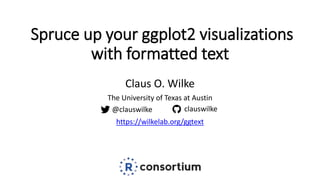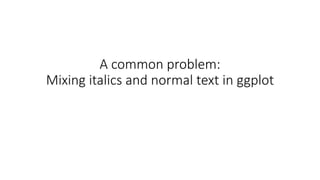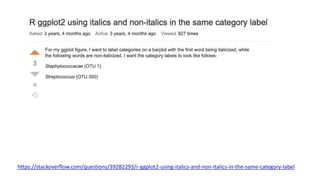Successfully reported this slideshow.

# Spruce up your ggplot2 visualizations with formatted text

5

Share×
1 of 33
1 of 33

# Spruce up your ggplot2 visualizations with formatted text

5

Share

Talk given at rstudio::conf 2020, January 30, 2020.

Talk given at rstudio::conf 2020, January 30, 2020.

## More Related Content

### Related Books

Free with a 14 day trial from Scribd

See all

### Related Audiobooks

Free with a 14 day trial from Scribd

See all

### Spruce up your ggplot2 visualizations with formatted text

1. 1. Spruce up your ggplot2 visualizations with formatted text Claus O. Wilke The University of Texas at Austin https://wilkelab.org/ggtext @clauswilke clauswilke
2. 2. A common problem: Mixing italics and normal text in ggplot
3. 3. https://stackoverflow.com/questions/39282293/r-ggplot2-using-italics-and-non-italics-in-the-same-category-label
4. 4. bactname OTUname name value Staphylococcaceae OTU 1 Staphylococcaceae (OTU 1) -0.5 Moraxella OTU 2 Moraxella (OTU 2) 0.5 Streptococcus OTU 3 Streptococcus (OTU 3) 2.0 Acinetobacter OTU 4 Acinetobacter (OTU 4) 3.0
5. 5. bactname OTUname name value Staphylococcaceae OTU 1 Staphylococcaceae (OTU 1) -0.5 Moraxella OTU 2 Moraxella (OTU 2) 0.5 Streptococcus OTU 3 Streptococcus (OTU 3) 2.0 Acinetobacter OTU 4 Acinetobacter (OTU 4) 3.0 data %>% ggplot(aes(name, value)) + geom_col() + coord_flip()
6. 6. bactname OTUname name value Staphylococcaceae OTU 1 Staphylococcaceae (OTU 1) -0.5 Moraxella OTU 2 Moraxella (OTU 2) 0.5 Streptococcus OTU 3 Streptococcus (OTU 3) 2.0 Acinetobacter OTU 4 Acinetobacter (OTU 4) 3.0 data %>% ggplot(aes(name, value)) + geom_col() + coord_flip()
7. 7. https://stackoverflow.com/questions/39282293/r-ggplot2-using-italics-and-non-italics-in-the-same-category-label
8. 8. How should we specify formatted text?
9. 9. data %>% ggplot(aes(name, value)) + geom_col() + coord_flip() + ggtitle("species names are *in italics*")
10. 10. data %>% ggplot(aes(name, value)) + geom_col() + coord_flip() + ggtitle("species names are *in italics*") library(ggtext) # remotes::install_github('wilkelab/ggtext')
11. 11. data %>% ggplot(aes(name, value)) + geom_col() + coord_flip() + ggtitle("species names are *in italics*") + theme(plot.title = element_markdown()) library(ggtext) # remotes::install_github('wilkelab/ggtext')
12. 12. data %>% mutate( name = glue("*{bactname}* ({OTUname})") # library(glue) )
13. 13. data %>% mutate( name = glue("*{bactname}* ({OTUname})") # library(glue) ) bactname OTUname name value Staphylococcaceae OTU 1 *Staphylococcaceae* (OTU 1) -0.5 Moraxella OTU 2 *Moraxella* (OTU 2) 0.5 Streptococcus OTU 3 *Streptococcus* (OTU 3) 2.0 Acinetobacter OTU 4 *Acinetobacter* (OTU 4) 3.0
14. 14. data %>% mutate( name = glue("*{bactname}* ({OTUname})") ) %>% ggplot(aes(name, value)) + geom_col() + coord_flip() + theme(axis.text.y = element_markdown())
15. 15. Your plots are boring. Don’t you ever use color?
16. 16. data %>% mutate( color = c("#009E73", "#D55E00", "#0072B2", "#000000"), name = glue("<i style='color:{color}'>{bactname}</i> ({OTUname})") ) %>%
17. 17. data %>% mutate( color = c("#009E73", "#D55E00", "#0072B2", "#000000"), name = glue("<i style='color:{color}'>{bactname}</i> ({OTUname})") ) %>% ggplot(aes(name, value, fill = color)) + geom_col(alpha = 0.5) + coord_flip() + scale_fill_identity() + theme(axis.text.y = element_markdown())
18. 18. What else can you do?
19. 19. https://stackoverflow.com/questions/14070953/photo- alignment-with-graph-in-r/30765590#30765590
20. 20. labels <- c( Interphase = "<img src='img/interphase.jpg' width='50' /><br>Interphase", Prophase = "<img src='img/prophase.jpg' width='50' /><br>Prophase", Metaphase = "<img src='img/metaphase.jpg' width='50' /><br>Metaphase", Anaphase = "<img src='img/anaphase.jpg' width='50' /><br>Anaphase", Telophase = "<img src='img/telophase.jpg' width='50' /><br>Telophase" ) https://stackoverflow.com/questions/14070953/photo- alignment-with-graph-in-r/30765590#30765590
21. 21. https://stackoverflow.com/questions/14070953/photo- alignment-with-graph-in-r/30765590#30765590 labels <- c( Interphase = "<img src='img/interphase.jpg' width='50' /><br>Interphase", ... ) ggplot(data, aes(phase, value, fill = cat)) + geom_col(position = "dodge") + scale_x_discrete(name = NULL, labels = labels) + theme(axis.text.x = element_markdown(lineheight = 1.2))
22. 22. https://stackoverflow.com/questions/14070953/photo- alignment-with-graph-in-r/30765590#30765590 labels <- c( Interphase = "<img src='img/interphase.jpg' width='50' /><br>Interphase", ... ) ggplot(data, aes(phase, value, fill = cat)) + geom_col(position = "dodge") + scale_x_discrete(name = NULL, labels = labels) + theme(axis.text.x = element_markdown(lineheight = 1.2))
23. 23. Text boxes, backgrounds, and word-wrapping https://wilkelab.org/ggtext
24. 24. Does this only work for theme elements?
25. 25. ggplot(iris, aes(Sepal.Length, Sepal.Width)) + geom_point() + geom_smooth(method = "lm") + facet_wrap(~Species)
26. 26. iris_cor <- iris %>% group_by(Species) %>% summarize(r_square = cor(Sepal.Length, Sepal.Width)^2) iris_cor #> # A tibble: 3 x 2 #> Species r_square #> <fct> <dbl> #> 1 setosa 0.551 #> 2 versicolor 0.277 #> 3 virginica 0.209
27. 27. iris_cor <- iris %>% group_by(Species) %>% summarize(r_square = cor(Sepal.Length, Sepal.Width)^2) %>% mutate( Sepal.Length = 8, Sepal.Width = 4.5, label = glue("*r*<sup>2</sup> = {round(r_square, 2)}") ) iris_cor #> # A tibble: 3 x 5 #> Species r_square Sepal.Length Sepal.Width label #> <fct> <dbl> <dbl> <dbl> <glue> #> 1 setosa 0.551 8 4.5 *r*<sup>2</sup> = 0.55 #> 2 versicolor 0.277 8 4.5 *r*<sup>2</sup> = 0.28 #> 3 virginica 0.209 8 4.5 *r*<sup>2</sup> = 0.21
28. 28. ggplot(iris, aes(Sepal.Length, Sepal.Width)) + geom_point() + geom_smooth(method = "lm") + geom_richtext( data = iris_cor, aes(label = label), hjust = 1, vjust = 1 ) + facet_wrap(~Species)
29. 29. The package that makes it all possible
30. 30. text <- "Some text **in bold.**" gp <- grid::gpar( fontfamily = "Comic Sans MS", fontsize = 20, col = "blue" ) g1 <- grid::textGrob(text, 0.1, 0.7, hjust = 0, gp = gp) grid::grid.draw(g1)
31. 31. text <- "Some text **in bold.**" gp <- grid::gpar( fontfamily = "Comic Sans MS", fontsize = 20, col = "blue" ) g1 <- grid::textGrob(text, 0.1, 0.7, hjust = 0, gp = gp) g2 <- gridtext::richtext_grob(text, 0.1, 0.4, hjust = 0, gp = gp) grid::grid.draw(g1) grid::grid.draw(g2)
32. 32. https://wilkelab.org/ggtext https://wilkelab.org/gridtext @clauswilke clauswilke